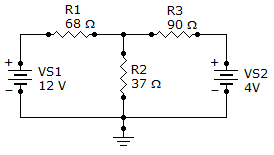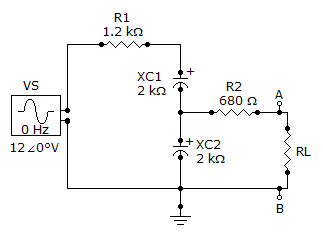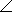# Online Electrical Engineering Test - Electrical Engineering Test 3Loading Test...

Instruction:

• This is a FREE online test. DO NOT pay money to anyone to attend this test.
• Total number of questions : 20.
• Time alloted : 30 minutes.
• Each question carry 1 mark, no negative marks.
• DO NOT refresh the page.
• All the best :-).

1.

A resistor is connected across a 50 V source. What is the current in the resistor if the color code is red, orange, orange, silver?

A.
 2 mAB.
 2.2 mAC.
 214 mAD.
 21.4 mA2.

What is the approximate resistance setting of a rheostat in which 650 mA of current flows with a 150 V source?

A.
 9.7B.
 97C.
 23D.
 2303.

The watt is the unit of power.

A.
 TrueB.
 False4.

The number of current paths equals the number of resistors in parallel.

A.
 TrueB.
 False5.

Total power in a parallel circuit is found by adding up the power of all the individual resistors.

A.
 TrueB.
 False6.

What is the voltage drop across R2?A.
 3.5 VB.
 4.18 VC.
 1.5 VD.
 145 mV7.

The first row of a certain determinant has the numbers 3 and 5. The second row has the numbers 7 and 2. The value of this determinant is

A.
 31B.
 –31C.
 39D.
 –298.

When the speed at which a conductor is moved through a magnetic field is increased, the induced voltage

A.
 increasesB.
 decreasesC.
 remains constantD.
 reaches zero9.

The induced voltage across a coil with 250 turns that is located in a magnetic field that is changing at a rate of 8 Wb/s is

A.
 1,000 VB.
 2,000 VC.
 31.25 VD.
 3,125 V10.

An electromagnetic field exists only when there is

A.
 an increasing currentB.
 decreasing currentC.
 voltageD.
 current11.

The average value of a 12 V peak sine wave over one complete cycle is

A.
 0 VB.
 1.27 VC.
 7.64 VD.
 6.37 V12.

Amplitude is the maximum value of a voltage or current.

A.
 TrueB.
 False13.

When the frequency is decreased, the impedance of a parallel RL circuit

A.
 increasesB.
 decreasesC.
 remains constantD.
 is not a factor14.

Referring to the given figure, determine ZTH as seen by RL if R1 is changed to 3.3 k.A.
 1488–70.7°B.
 3859–31.2°C.
 5180–50.5°D.
 1828–50.2°15.

Determine VTH if R1 is changed to 3.3 k.A.
 0.57416.7° VB.
 4.6316.7° VC.
 4.6339.5° VD.
 0.46339.5° V16.

One circuit is equivalent to another, in the context of Thevenin's theorem, when the circuits produce the same voltage.

A.
 TrueB.
 False17.

In an RC differentiator, the capacitor

A.
 charges exponentially at a rate depending on the RC time constantB.
 charges exponentially at a rate depending on the input voltageC.
 charges when the input voltage is decreasingD.
 charges to approximately one time constant18.

If in a Y-connected ac generator, each phase voltage has a magnitude of 90 VRMS, what is the magnitude of each line voltage?

A.
 0 VB.
 90 VC.
 156 VD.
 180 V19.

A simple three-phase generator consists of three conductive loops separated by 120°.

A.
 TrueB.
 False20.

The stator is the rotating assembly in a generator or motor.

A.
 TrueB.
 FalseExplanation:

The two main parts of a generator or motor can be described in either mechanical or electrical terms:

Mechanical:

Rotor: The rotating part of an electrical machine
Stator: The stationary part of an electrical machine

Electrical:

Armature: The power-producing component of an electrical machine. In a generator, alternator, or dynamo the armature windings generate the electric current. The armature can be on either the rotor or the stator.
Field: The magnetic field component of an electrical machine. The magnetic field of the dynamo or alternator can be provided by either electromagnets or permanent magnets mounted on either the rotor or the stator.